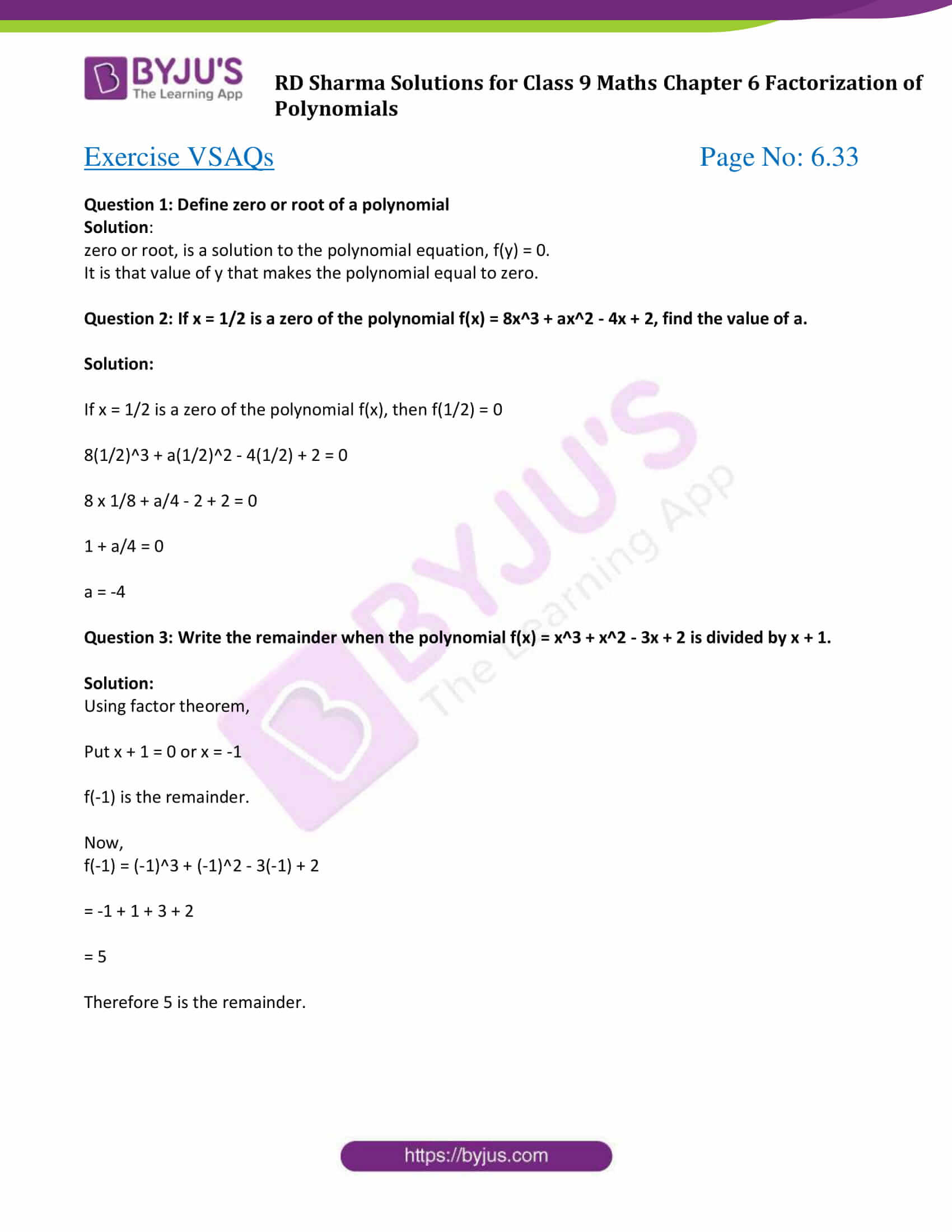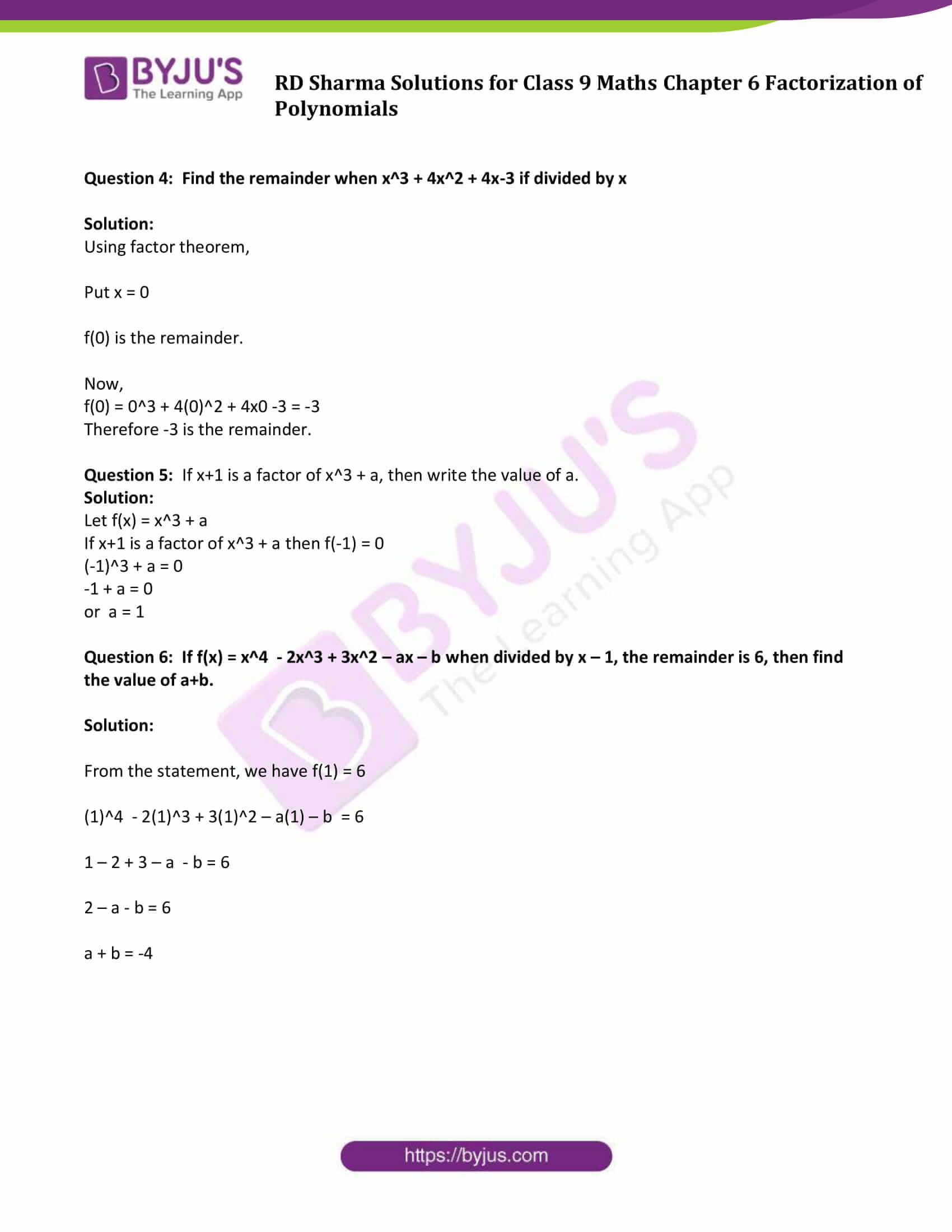# RD Sharma Solutions for Class 9 Maths Chapter 6 Factorization of Polynomials Exercise VSAQs

RD Sharma Class 9 Mathematics Chapter 6 Exercise VSAQs Factorization of Polynomials is provided here. In this exercise, students will learn how to perform factorisation of a polynomial using different methods. RD Sharma solutions for class 9 students to help you in your endeavour to have a better understanding of factorization of Polynomials.

## Download PDF of RD Sharma Solutions for Class 9 Maths Chapter 6 Factorization of Polynomials Exercise VSAQs### Access Answers to Maths RD Sharma Solutions for Class 9 Chapter 6 Factorization of Polynomials Exercise VSAQs Page number 6.33

#### Exercise VSAQs Page No: 6.33

Question 1: Define zero or root of a polynomial

Solution:

zero or root, is a solution to the polynomial equation, f(y) = 0.

It is that value of y that makes the polynomial equal to zero.

Question 2: If x = 1/2 is a zero of the polynomial f(x) = 8x3 + ax2 – 4x + 2, find the value of a.

Solution:

If x = 1/2 is a zero of the polynomial f(x), then f(1/2) = 0

8(1/2)3 + a(1/2)2 – 4(1/2) + 2 = 0

8 x 1/8 + a/4 – 2 + 2 = 0

1 + a/4 = 0

a = -4

Question 3: Write the remainder when the polynomial f(x) = x3 + x2 – 3x + 2 is divided by x + 1.

Solution:

Using factor theorem,

Put x + 1 = 0 or x = -1

f(-1) is the remainder.

Now,

f(-1) = (-1)3 + (-1)2 – 3(-1) + 2

= -1 + 1 + 3 + 2

= 5

Therefore 5 is the remainder.

Question 4: Find the remainder when x3 + 4x2 + 4x-3 if divided by x

Solution:

Using factor theorem,

Put x = 0

f(0) is the remainder.

Now,

f(0) = 03 + 4(0)2 + 4×0 -3 = -3

Therefore -3 is the remainder.

Question 5: If x+1 is a factor of x3 + a, then write the value of a.

Solution:

Let f(x) = x3 + a

If x+1 is a factor of x3 + a then f(-1) = 0

(-1)3 + a = 0

-1 + a = 0

or a = 1

Question 6: If f(x) = x^4 – 2x3 + 3x2 – ax – b when divided by x – 1, the remainder is 6, then find the value of a+b.

Solution:

From the statement, we have f(1) = 6

(1)4 – 2(1)3 + 3(1)2 – a(1) – b = 6

1 – 2 + 3 – a – b = 6

2 – a – b = 6

a + b = -4

## RD Sharma Solutions for Class 9 Maths Chapter 6 Factorization of Polynomials Exercise VSAQs

RD Sharma Solutions Class 9 Maths Chapter 6 Factorization of Polynomials Exercise VSAQs is based on remainder Theorem, factor Theorem and factorisation of polynomials by using the factor theorem. Exercise VSAQs will help students to understand the important steps involved in factorization using different methods.